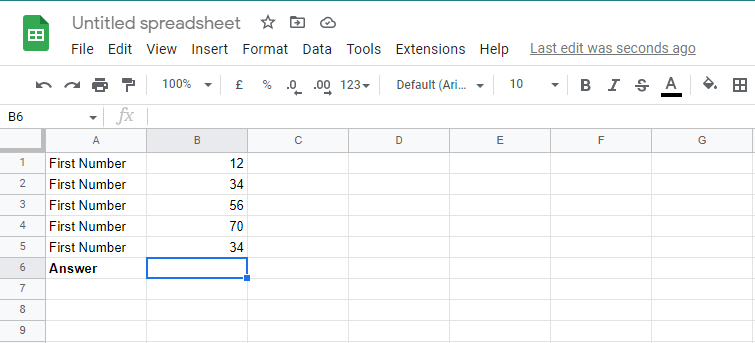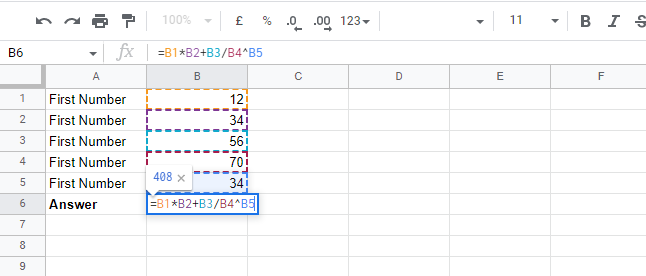# How to create a complex formula using the order of operations in Google Sheets?

Published June 12, 2022

Google sheets is a robust application that enables you to solve complex mathematical problems such as calculating taxes, compound interests, the area of a histogram etc. you can easily create a complex formula that meets your needs.   However, to create a complex formula that completely works based on your needs, you definitely need to learn how Google sheet processes the complex formulas. Google Sheets uses the order of operations to determine which operation to perform first when calculating these complexes. In this article, we are going to learn:

• How the order of operation works

• How to create a complex formula using the order of operations in Google sheets.

## How the order of operation works

Google Sheets processes complex formulas using the "order of operations" principle. The following sequence of operations is followed by Google Sheets when processing complex formulas.

• First, the operations in the enclosed parentheses

• Then, executes the exponential calculations

• Then executes division and multiplication, whichever comes first

• Lastly executes subtraction and addition. Whichever comes first.

Let's see now how we can create a complex formula using the order operations in Google Sheets.

## How to create complex formulas using order of operations

It is remarkably easy to create complex formulas. All you have to do is note the mathematical operators, values, and cell references to be used. In this article, we will create our formula using four mathematical operators.

Step 1:  First of all, select the cell that will contain the formula.Step 2: Next, enter your complex formula in the selected cell. To do so, the first type equals "=" and then adds the formula. In this case, we will add the formula "=B1*B2+B3/B4^B5"Step 3: Now, press enter on your keyboard to generate the answer.First, the formula calculates the exponential formula "B4*B5", then it processes the multiplication "B1*B2", then the division "B3/B4*B5", and finally, it performs the addition "B1*B2 + (B3/B4*B5)" to arrive at the answer.

Conclusion

We now know how to create complex formulas and how to use the order of operations to process them on the Google sheet.  In any case, it is always a good idea to double-check your formula since Google Sheets won't let you know if your formula contains errors

 Article Contributed By :155 Views
Projects By category
Categories